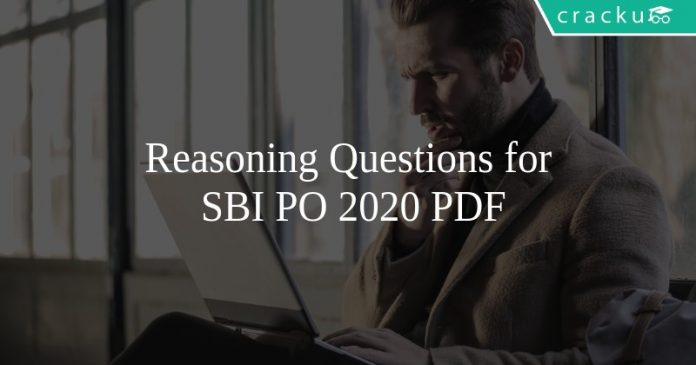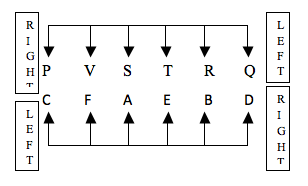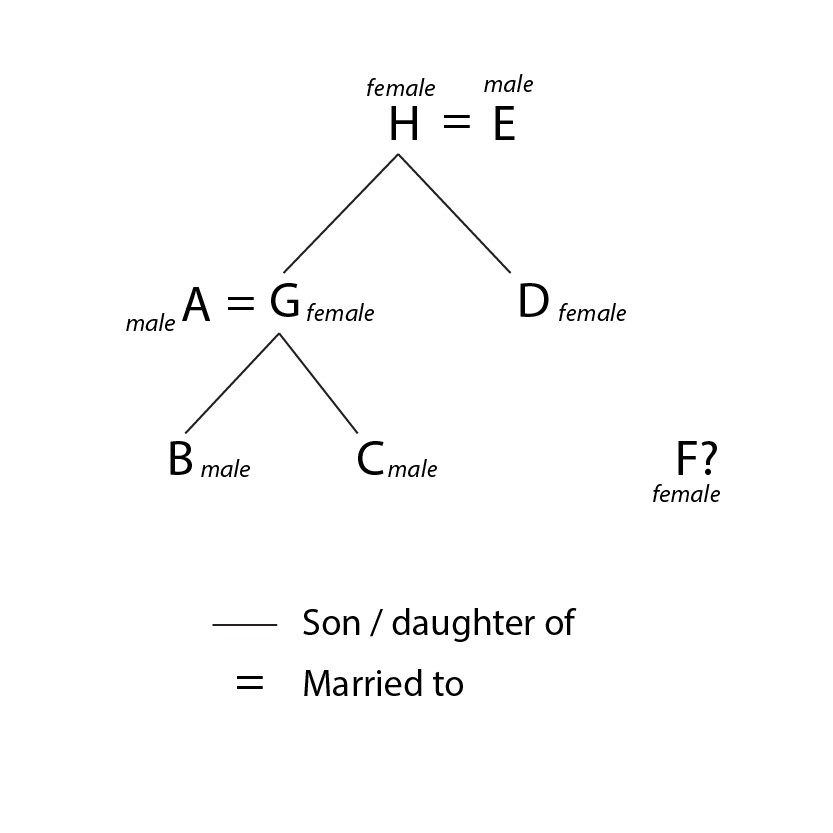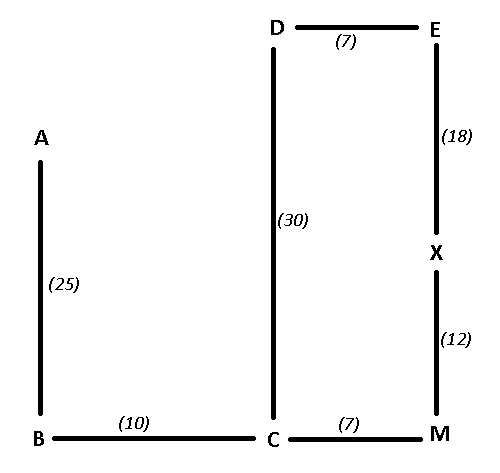# Reasoning Questions for SBI PO 2020 PDF

0
696Reasoning Questions for SBI PO 2020 PDF

# Reasoning Questions for SBI PO 2020 pdf

Download SBI PO Reasoning Questions & Answers PDF for SBI PO Prelims and Mains exam. Top-15 Very Important SBI PO Questions with solutions for Banking Exams.

Instructions

Each of the following question consists of a question and two statements numbered I and II given below it.You have to decide whether the data provided the statement are sufficient to answer the question Read the statements and Give answer

a:If the data in Statement I alone are sufficient to answer the question while the data in Statement II alone are not Sufficient to answer the question
b:If the data in Statement II alone are sufficient to answer the question While the data in Statement I alone are not Sufficient to answer the question
c:If the data either in Statement I alone or in Statement II alone are sufficient to answer the question
d:If the data in both the statements I and II together are not Sufficient to answer the question
e:If the data in both the Statements I and II together are necessary to answer the question

Question 1: Village P is towards which directions of village R ?
I. Village R is to the South-East of village T and T is to the North of village P.
II. Village Q is the South of village P and to the South-West of village R.

a) If the data in Statement I alone are sufficient to answer the question while the data in Statement II alone are not Sufficient to answer the question

b) If the data in Statement II alone are sufficient to answer the question While the data in Statement I alone are not Sufficient to answer the question

c) If the data either in Statement I alone or in Statement II alone are sufficient to answer the question

d) If the data in both the statements I and II together are not Sufficient to answer the question

e) If the data in both the Statements I and II together are necessary to answer the question

Question 2: Who among A,B,C, D and E, each having a different heights is the third tallest ?
I.E is shorter than only B
II. C is taller than only A

a) If the data in Statement I alone are sufficient to answer the question while the data in Statement II alone are not Sufficient to answer the question

b) If the data in Statement II alone are sufficient to answer the question While the data in Statement I alone are not Sufficient to answer the question

c) If the data either in Statement I alone or in Statement II alone are sufficient to answer the question

d) If the data in both the statements I and II together are not Sufficient to answer the question

e) If the data in both the Statements I and II together are necessary to answer the question

Instructions

Instructions: Study the information and answer the questions given questions:
Twelve people are sitting in two parallel rows containing six people each, in such a way that there is an equal distance between adjacent persons. In row-1 P, Q, R, S, T and V are seated and all of them are facing South. In Row – 2: A, B, C, D, E and F are seated and all of them are facing North. Therefore, in the given seating arrangement each member seated in a row faces another member of the other row.
S sits third to right of Q. Either S or Q sits at an extreme end of the line. The one who faces Q sits second to right of E. Two people sit between B and F. Neither B nor F sits at an extreme end of the line. The immediate neighbor of B faces the person who sits third to left of P, R and T are immediate neighbours of each other. C sits second to the left of A. T does not face the immediate neighbor of D.

Question 3: Who amongst the following sit at extreme ends of the rows?

a) S, D

b) Q, A

c) V, C

d) P, D

e) Q, F

Question 4: Who amongst the following faces S?

a) A

b) B

c) C

d) D

e) F

SBI PO Free Mock Tests

Question 5: How many persons are seated between V and R?

a) One

b) Two

c) Three

d) Four

e) None

Question 6: P is related to A in the same way as S is related to B based on the given arrangement. To which of the following is T related to, following same pattern?

a) C

b) D

c) E

d) F

e) Cannot determined

Question 7: Which of the following is true regarding T?

a) F faces T

b) V is an immediate neighbour of T

c) F faces the one who is second to right of T

d) T sits at one of the extreme ends of the line

e) Q sits second to the right of T

Instructions

In a family of 8 people, G is the mother of B. H has two daughters – G and D. F is H’s only granddaughter. E is D’s father. C is the brother of B. B is A’s son.

Question 8: How many children does D have?

a) 0

b) 1

c) 2

d) Either 0 or 1

e) Either 1 or 2

Question 9: How is D related to C?

a) Uncle

b) Aunt

c) Brother

d) Sister

e) Can’t be determined

Instructions

Read the given information carefully and answer the given questions. B is 25 m south of A. C is 10 m east of B. D is 30 m north of C. E is 7 m east of D. X is 18 m south of E. M is 12 m south of X. C is 7 m west of M.

Question 10: B is in which direction from Point D ?

a) South

b) South­West

c) North­East

d) South­East

e) North

Question 11: If Point W is 3 m to the north of A, then what is the distance between B and W ?

a) 28 m

b) 15 m

c) 21 m

d) 24 m

e) 17 m

Question 12: What is the distance between B and M?

a) 17 m

b) 15 m

c) 21 m

d) 19 m

e) 13 m

Instructions

Study the following arrangement carefully and answer the questions given below :
1 5 8 4 2 1 5 2 3 4 5 6 7 8 9 5 1 4 1 5 6 8 7 4

Question 13: How many such 1s are there in the above arrangement each of which is immediately followed by a perfect square ?

a) None

b) One

c) Two

d) Three

e) More than three

Question 14: How many such 4s are there in the above arrangement each of which is immediately preceded by a digit which has a numerical value more than four ?

a) None

b) One

c) Two

d) Three

e) More than three

Question 15: If all the even digits are deleted from the above arrangement which of the following will be tenth from the right end of the arrangement ?

a) 5

b) 3

c) 1

d) 7

e) 9

Answers & Solutions:

1) Answer (D)

I : Village R is south east of Village T and there is no specific information about position of village P.

Thus, I alone is insufficient.

Similarly, in II, there is no specific information about position of R.

Thus, II alone is insufficient.

I & II : We know, that T is north of P and Q is south of P. Thus, T, P & Q are in a straight vertical line in that order.

There is still not specific info about position of P. P can be to the west or north-west or south-west of R.

Thus, I & II together are not sufficient.

2) Answer (E)

I : E is shorter than only B.

=> B is the tallest and E is the second tallest. => B > E > A, C, D.

Since, we can’t find the third tallest, I alone is insufficient.

II : C is taller than only A.

=> A is the shortest and C is the fourth tallest. => B,D,E > C > A

Since, we can’t find the third tallest, II alone is insufficient.

I & II : From I and II, we get

B > E > D > C > A.

=> D is the third tallest.

Thus, I & II together are sufficient.

3) Answer (D)

Since one of S and Q sit at the extreme, Q has to sit at the right end and S three places to the right of him. Now that Q is fixed, we can place E as shown in the figure. B and F can’t be at extreme and 2 people are sitting between them. Also, B faces the person who sits third to left of P. Thus, B, F and P can only be placed as shown in the figure. Now C sits second to the left of A. So, C is at the extreme and A second to the right of him. This means D is at the rightmost corner. So, R must sit adjacent to Q and T adjacent to R. The arrangement is as shown below-P and D sit at extreme ends of the rows

4) Answer (A)

Since one of S and Q sit at the extreme, Q has to sit at the right end and S three places to the right of him. Now that Q is fixed, we can place E as shown in the figure. B and F can’t be at extreme and 2 people are sitting between them. Also, B faces the person who sits third to left of P. Thus, B, F and P can only be placed as shown in the figure. Now C sits second to the left of A. So, C is at the extreme and A second to the right of him. This means D is at the rightmost corner. So, R must sit adjacent to Q and T adjacent to R. The arrangement is as shown below-A faces S.

5) Answer (B)

Since one of S and Q sit at the extreme, Q has to sit at the right end and S three places to the right of him. Now that Q is fixed, we can place E as shown in the figure. B and F can’t be at extreme and 2 people are sitting between them. Also, B faces the person who sits third to left of P. Thus, B, F and P can only be placed as shown in the figure. Now C sits second to the left of A. So, C is at the extreme and A second to the right of him. This means D is at the rightmost corner. So, R must sit adjacent to Q and T adjacent to R. The arrangement is as shown below-Two persons – S and T – are seating between V and R.

6) Answer (B)

Since one of S and Q sit at the extreme, Q has to sit at the right end and S three places to the right of him. Now that Q is fixed, we can place E as shown in the figure. B and F can’t be at extreme and 2 people are sitting between them. Also, B faces the person who sits third to left of P. Thus, B, F and P can only be placed as shown in the figure. Now C sits second to the left of A. So, C is at the extreme and A second to the right of him. This means D is at the rightmost corner. So, R must sit adjacent to Q and T adjacent to R. The arrangement is as shown below-P faces the person who sits second to the left of A. S faces the person who sits second to the left of B. Similarly, T faces E who sits second to the left of D.

7) Answer (C)

Since one of S and Q sit at the extreme, Q has to sit at the right end and S three places to the right of him. Now that Q is fixed, we can place E as shown in the figure. B and F can’t be at extreme and 2 people are sitting between them. Also, B faces the person who sits third to left of P. Thus, B, F and P can only be placed as shown in the figure. Now C sits second to the left of A. So, C is at the extreme and A second to the right of him. This means D is at the rightmost corner. So, R must sit adjacent to Q and T adjacent to R. The arrangement is as shown below-F faces V who sits second to the right of T.

8) Answer (D)

From the information given, we can draw a family tree as shown below.We don’t know if F is G’s or D’s daughter. Hence, D has either 0 or 1 child.

9) Answer (B)

From the information given, we can draw a family tree as shown below.Hence, D is C’s Aunt.

10) Answer (B)

Based on the above conditions, the points when plotted on a graph will be :

where the numbers in the brackets is the distance between two points.B is in south-west direction from point D.

Thus, Ans – (B)

11) Answer (A)

Based on the above conditions, the points when plotted on a graph will be :

where the numbers in the brackets is the distance between two points.If we, point W is 3 m to the north of point A

Distance between W and B = 3+25 = 28.

Thus, Ans – (A)

12) Answer (A)

Based on the above conditions, the points when plotted on a graph will be :

where the numbers in the brackets is the distance between two points.As seen from above, distance between B and M = 10+7 = 17

Thus, Ans – (A)

13) Answer (B)

Arrangement : 1 5 8 4 2 1 5 2 3 4 5 6 7 8 9 5 1 4 1 5 6 8 7 4

1’s which are immediately followed by a perfect square

= (1) (perfect square)

= 1 5 8 4 2 1 5 2 3 4 5 6 7 8 9 5 1 4 1 5 6 8 7 4

Thus, there is only one such 1.

=> Ans – (B)

14) Answer (C)

Arrangement : 1 5 8 4 2 1 5 2 3 4 5 6 7 8 9 5 1 4 1 5 6 8 7 4

4’s which are immediately preceded by a digit which has a numerical value more than four = (>4) (4)

= 1 5 8 4 2 1 5 2 3 4 5 6 7 8 9 5 1 4 1 5 6 8 7 4

Thus, there are two such 4’s.

=> Ans – (C)

15) Answer (A)

Arrangement : 1 5 8 4 2 1 5 2 3 4 5 6 7 8 9 5 1 4 1 5 6 8 7 4

If all the even digits are deleted, then

= 1 5 1 5 3 5 7 9 5 1 1 5 7

10th from right end = 5

=> Ans – (A)

We hope this Reasoning Questions & Answers  PDF for SBI PO Exams preparation is so helpful to you.